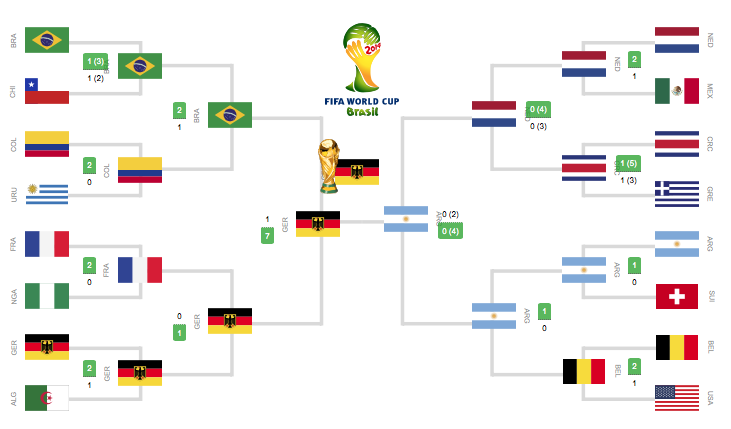Probabilitical PlayersSixteen players $S_{1}, S_{2}, ..., S_{16}$ play in a tournament. They are divided into eight pairs at random. From each pair a winner is decided on the basis of a game played between the two players of the pair. Assume that all the players are of equal strength.

Find the probability that exactly one of the two players $S_{1}$ and $S_{2}$ is among the eight winners.

The probability is in the form $\frac{a}{b}$. Find a + b.

To try more such problems click here.

×